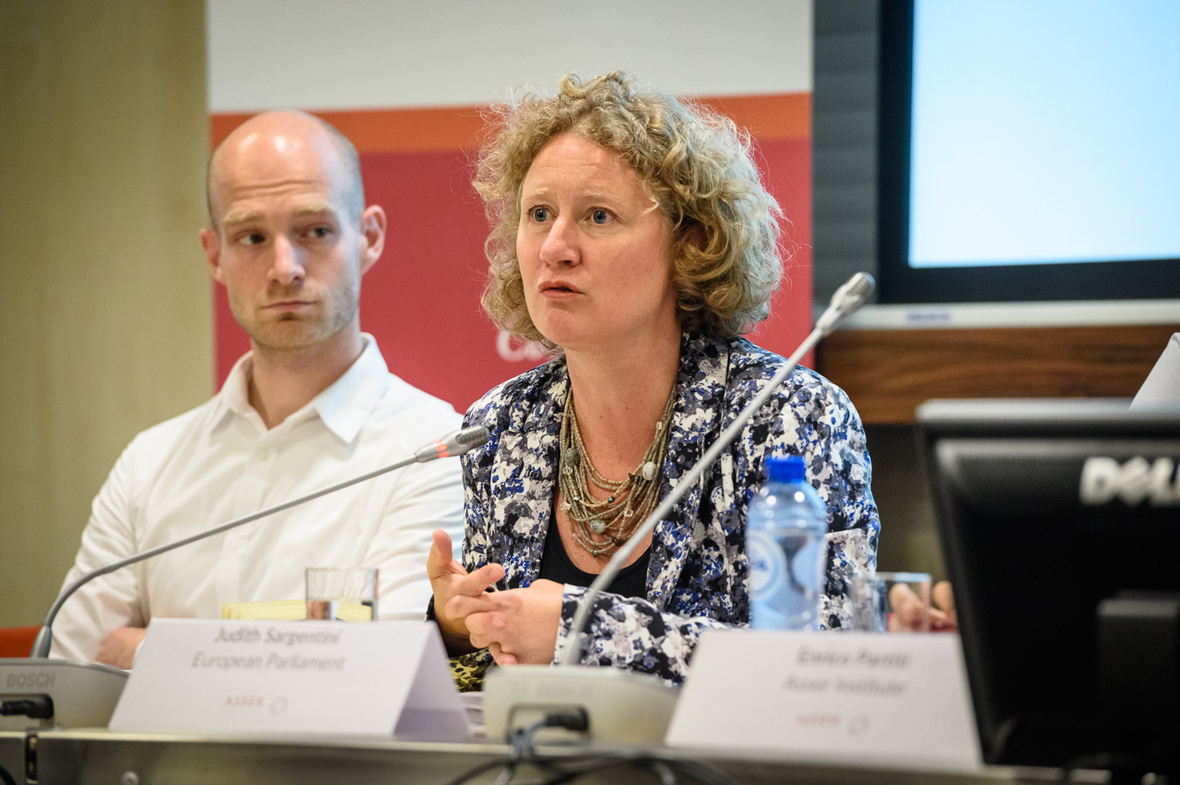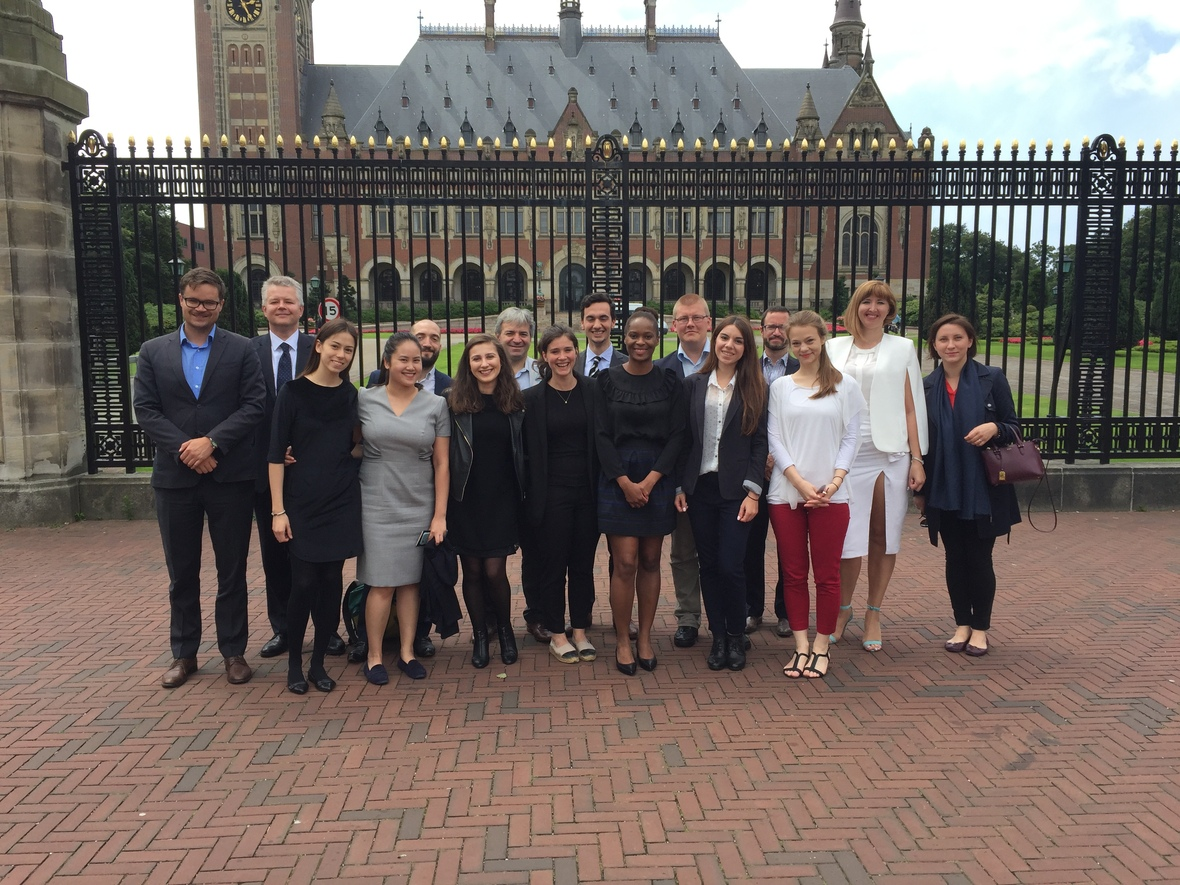Like   Tweet   in

# Asser Today September 2017

 table div table+table+table div table{width:100%;padding:0}table div table+table+table div table img{width:96.23%;padding:0;float:none}table div table+table+table div table td{width:100%;padding:0 1.88% 18px}/* styles */# Research

## Selected Publications

▪ Uladzislau Belavusau and Aleksandra Gliszczynska-Grabias, Memory Laws: Mapping a New Subject in Comparative Law and Transitional Justice, SSRN Asser Research Paper. Forthcoming in Law and Memory.
 ▪ Uladzislau Belavusau and Aleksandra Gliszczynska-Grabias, Memory Laws: Mapping a New Subject in Comparative Law and Transitional Justice, SSRN Asser Research Paper. Forthcoming in Law and Memory.

▪ Eva Kassoti, Trading with Settlements: The International Obligations of the European Union with Regard to Economic Dealings with Occupied Territories. SSRN Asser Research Paper.
 ▪ Eva Kassoti, Trading with Settlements: The International Obligations of the European Union with Regard to Economic Dealings with Occupied Territories. SSRN Asser Research Paper.

▪ Enrico Partiti and Steffen van der Velde, The Promotion of Sustainable Development through EU Trade Instruments. SSRN Asser Research Paper. Forthcoming in Journal of World Trade 51 (6).
 ▪ Enrico Partiti and Steffen van der Velde, The Promotion of Sustainable Development through EU Trade Instruments. SSRN Asser Research Paper. Forthcoming in Journal of World Trade 51 (6).

Asser research papers and policy briefs can be downloaded on SSRN.

## Research news# First court cases show: EU Timber Regulation starts to work# Getting to a Responsible Garment Industry# New ILA Study Group on The Role of Cities in International Law

 /* styles */
 table div table+table+table+table+table+table+table+table+table+table div table{width:100%;padding:0}table div table+table+table+table+table+table+table+table+table+table div table img{width:96.23%;padding:0;float:none}table div table+table+table+table+table+table+table+table+table+table div table td{width:100%;padding:0 1.88% 18px}/* styles */# Education and Events

The Asser Institute has extensive experience in delivering postgraduate training programmes and in knowledge dissemination in the field of international and European law.

## Upcoming

 table div table+table+table+table+table+table+table+table+table+table+table+table div table{width:100%;padding:0}table div table+table+table+table+table+table+table+table+table+table+table+table div table img{width:96.23%;padding:0;float:none}table div table+table+table+table+table+table+table+table+table+table+table+table div table td{width:100%;padding:0 1.88% 18px}/* styles */table div table+table+table+table+table+table+table+table+table+table+table+table+table div table,table.module-12{width:35.66%;float:left;padding:0}table div table+table+table+table+table+table+table+table+table+table+table+table+table div table a{border:0 none;text-decoration:none}table div table+table+table+table+table+table+table+table+table+table+table+table+table div table img{width:100%!important;border:0 none;text-decoration:none}table div table+table+table+table+table+table+table+table+table+table+table+table+table div table td{width:100%;padding:0 20px 20px 0}/* styles */
 table div table+table+table+table+table+table+table+table+table+table+table+table+table+table div table{width:100%;padding:0}table div table+table+table+table+table+table+table+table+table+table+table+table+table+table div table img{width:96.23%;padding:0;float:none}table div table+table+table+table+table+table+table+table+table+table+table+table+table+table div table td{width:100%;padding:0 1.88% 18px}/* styles */The Amsterdam Center for International Law (ACIL) and the T.M.C. Asser Instituut invited Professor Drumbl to lecture about the relationship between and roles of victims and perpetrators under the title of 'Tragic Perpetrators and Imperfect Victims'.

More information and registration

 table div table+table+table+table+table+table+table+table+table+table+table+table+table+table+table+table div table{width:100%;padding:0}table div table+table+table+table+table+table+table+table+table+table+table+table+table+table+table+table div table img{width:96.23%;padding:0;float:none}table div table+table+table+table+table+table+table+table+table+table+table+table+table+table+table+table div table td{width:100%;padding:0 1.88% 18px}/* styles */ISLJ Annual International Sports Law Conference, with contributions of Miguel Maduro and Richard McLaren.

More information on the conference website.

 table div table+table+table+table+table+table+table+table+table+table+table+table+table+table+table+table+table+table div table{width:100%;padding:0}table div table+table+table+table+table+table+table+table+table+table+table+table+table+table+table+table+table+table div table img{width:96.23%;padding:0;float:none}table div table+table+table+table+table+table+table+table+table+table+table+table+table+table+table+table+table+table div table td{width:100%;padding:0 1.88% 18px}/* styles */## HighlightFrom 10 - 14 July 2017, 18 participants travelled to The Hague to participate in Summer School on The Law and Practice of International Dispute Settlement. The Summer School is a collaboration between the Amsterdam Center for International Law (ACIL) of the University of Amsterdam and the T.M.C. Asser Instituut.

 table div table+table+table+table+table+table+table+table+table+table+table+table+table+table+table+table+table+table+table+table+table div table{width:100%;padding:0}table div table+table+table+table+table+table+table+table+table+table+table+table+table+table+table+table+table+table+table+table+table div table img{width:96.23%;padding:0;float:none}table div table+table+table+table+table+table+table+table+table+table+table+table+table+table+table+table+table+table+table+table+table div table td{width:100%;padding:0 1.88% 18px}/* styles */table.module-21{width:43.21%;padding:0}table div table+table+table+table+table+table+table+table+table+table+table+table+table+table+table+table+table+table+table+table+table+table div table{width:43.21%;float:none;margin-left:auto;margin-right:auto;padding:0}table div table+table+table+table+table+table+table+table+table+table+table+table+table+table+table+table+table+table+table+table+table+table div table a{border:0 none;text-decoration:none}table div table+table+table+table+table+table+table+table+table+table+table+table+table+table+table+table+table+table+table+table+table+table div table img{width:100%!important;border:0 none;text-decoration:none}table div table+table+table+table+table+table+table+table+table+table+table+table+table+table+table+table+table+table+table+table+table+table div table td{width:100%;padding:0}/* styles */
 table div table+table+table+table+table+table+table+table+table+table+table+table+table+table+table+table+table+table+table+table+table+table+table div table{width:100%;padding:0}table div table+table+table+table+table+table+table+table+table+table+table+table+table+table+table+table+table+table+table+table+table+table+table div table img{width:96.23%;padding:0;float:none}table div table+table+table+table+table+table+table+table+table+table+table+table+table+table+table+table+table+table+table+table+table+table+table div table td{width:100%;padding:0 1.88% 18px}/* styles */table div table+table+table+table+table+table+table+table+table+table+table+table+table+table+table+table+table+table+table+table+table+table+table+table div table,table.module-23{width:32.08%;float:left;padding:0}table div table+table+table+table+table+table+table+table+table+table+table+table+table+table+table+table+table+table+table+table+table+table+table+table div table a{border:0 none;text-decoration:none}table div table+table+table+table+table+table+table+table+table+table+table+table+table+table+table+table+table+table+table+table+table+table+table+table div table img{width:100%!important;border:0 none;text-decoration:none}table div table+table+table+table+table+table+table+table+table+table+table+table+table+table+table+table+table+table+table+table+table+table+table+table div table td{width:100%;padding:0 20px 20px 0}/* styles */ Print ISBN 978-94-6265-191-3 EBook/Online ISBN: 978-94-6265-192-0 XVIII+305 p., hardcover, EUR 139.99
 /* styles */
 table div table+table+table+table+table+table+table+table+table+table+table+table+table+table+table+table+table+table+table+table+table+table+table+table+table+table div table{width:100%;padding:0}table div table+table+table+table+table+table+table+table+table+table+table+table+table+table+table+table+table+table+table+table+table+table+table+table+table+table div table img{width:96.23%;padding:0;float:none}table div table+table+table+table+table+table+table+table+table+table+table+table+table+table+table+table+table+table+table+table+table+table+table+table+table+table div table td{width:100%;padding:0 1.88% 18px}/* styles */# Newsletter of the T.M.C. Asser Instituut

Editors:
Ruud Stevens/Antoinette Wessels/Faten Bushehri

The T.M.C. Asser Instituut carries out research on developments in international and European law and its potential for serving the cultivation of trust and respect in the global, regional, national and local societies in which the law operates.

 table div table+table+table+table+table+table+table+table+table+table+table+table+table+table+table+table+table+table+table+table+table+table+table+table+table+table+table+table div table{width:100%;padding:0}table div table+table+table+table+table+table+table+table+table+table+table+table+table+table+table+table+table+table+table+table+table+table+table+table+table+table+table+table div table img{width:96.23%;padding:0;float:none}table div table+table+table+table+table+table+table+table+table+table+table+table+table+table+table+table+table+table+table+table+table+table+table+table+table+table+table+table div table td{width:100%;padding:0 1.88% 18px}/* styles */